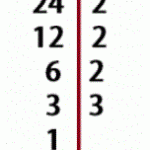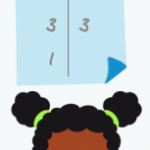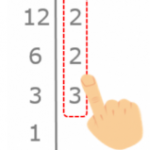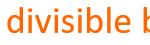Smartick is a fun way to learn math!# I want to learn about: Divisibility

Content about divisibility in elementary mathematics. Here you will find information about divisibility adapted for elementary aged children to make learning mathematics easier and more fun.

Apr06

## The Reason for Divisibility CriteriaSometimes we may be interested in knowing if one number is divisible by another, without having to perform a division. This is especially useful when we work with large numbers that require a lot of time for completing calculations. If you would like to refresh your memory on divisibility criteria, take a look at this […]

Sep02

## What is Factorial Decomposition?In today’s post we are going to answer the question, what is factorial decomposition? I hope that by the end of the post you’ll have it crystal clear. Definition of Factorial Decomposition We are going to start by remembering what a ”factor” is in mathematics. A factor is every number that is multiplied by another. […]

Dec31

## Divisibility by 4: How Do We Know If a Number Is Divisible by 4?In this post, we are going to learn the criteria for divisibility of the number 4 and understand how they function. The divisibility criteria for the number 4 are rules to know if a number can be divided by 4. They are simple to learn and their explanations are easy to understand. How do we […]

Dec24

## Some Prime Number CuriositiesFor this entry, we are going to learn some surprising facts about prime numbers. We would recommend reading our post about prime numbers and composite numbers first, to refresh your knowledge about these numbers. Are you ready to move on? Perfect! I’m sure that you know it is possible to write some numbers as products of […]

Jul23

## Explanation of the Formula to Calculate the Least Common MultipleI am sure this sounds familiar to many of you: To calculate the least common multiple (LCM), we have to choose between the common and uncommon factors that have the highest exponent. But what does it mean? Why do we have to choose from all factors…and out of the common factors, why the ones with […]

Sep29

## Follow 8 Easy Steps to Factor a NumberIn this entry, we’re going to explain how to break down or factor a number to its prime numbers step by step. Before we start anything, feel free to look at these entries where we explain what “factoring” means and what a prime number is so you can refresh your memory. Factorization How to factor […]

Aug07

## Divisibility Guidelines for 5 and Some ExamplesIn this post we will learn the criteria of divisibility by 5. A number is divisible by 5 when dividing the number by 5 results in a remainder of zero. But how can we know without having to complete the division? Here we show you the easiest way.   Divisibility criteria for 5: To determine if a number is […]

Jun07

## Follow the Divisibility Guidelines for 3In the post for this week, I will explain the criteria for the divisibility of 3. When they ask you “Is this number divisible by 3?”  The most intuitive thing to do is to divide that number by 3, and if the remainder is zero then the number itself is divisible by 3. For example: One […]